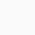👤 Join WhatsApp Learners
Deal Score+1

Calculus Applied: Apply tools of single-variable calculus to create and analyze mathematical models used by real practitioners in social, life, and physical sciences.

### Join Email Learners

Follow the guide on landing page

In this course, we go beyond the calculus textbook, working with practitioners in social, life, and physical sciences to understand how calculus and mathematical models play a role in their work.

Through a series of case studies, you’ll learn:

• How standardized test makers use functions to analyze the difficulty of test questions;
• How economists model the interaction of price and demand using rates of change, in a historical case of subway ridership;
• How an x-ray is different from a CT-scan, and what this has to do with integrals;
• How biologists use differential equation models to predict when populations will experience dramatic changes, such as extinction or outbreaks;
• How the Lotka-Volterra predator-prey model was created to answer a biological puzzle;
• How statisticians use functions to model data, like income distributions, and how integrals measure chance;
• How Einstein’s Energy Equation, E=mc2 is an approximation to a more complicated equation.

With real practitioners as your guide, you’ll explore these situations in a hands-on way: looking at data and graphs, writing equations, doing calculus computations, and making educated guesses and predictions.

This course provides a unique supplement to a course in single-variable calculus. Key topics include the application of derivatives, integrals and differential equations, mathematical models and parameters.

This course is for anyone who has completed or is currently taking a single-variable calculus course (differential and integral), at the high school (AP or IB) or college/university level. You will need to be familiar with the basics of derivatives, integrals, and differential equations, as well as functions involving polynomials, exponentials, and logarithms.

This is a course to learn applications of calculus to other fields, and NOT a course to learn the basics of calculus. Whether you’re a student who has just finished an introductory Calculus course or a teacher looking for more authentic examples for your classroom, there is something for you to learn here, and we hope you’ll join us!

• Authentic examples and case studies of how calculus is applied to problems in other fields
• How to analyze mathematical models, including variables, constants, and parameters
• Appreciation for the assumptions and complications that go into modeling real-world situations with mathematics
1DISCLAIMER: Courses on Future Syllabus are free but subject to return to their original prices on host platforms upon coupon expiration. Enrol while they are free.#### Silas Bamigbola

Certified Computer Engineer & Author, 'Lost Boys'. I believe in the influence of right information. Join me on Twitter.

Stay active
error: Content is protected !!Register New Account
Login/Register via your social accounts (fully secured)

or proceed manually